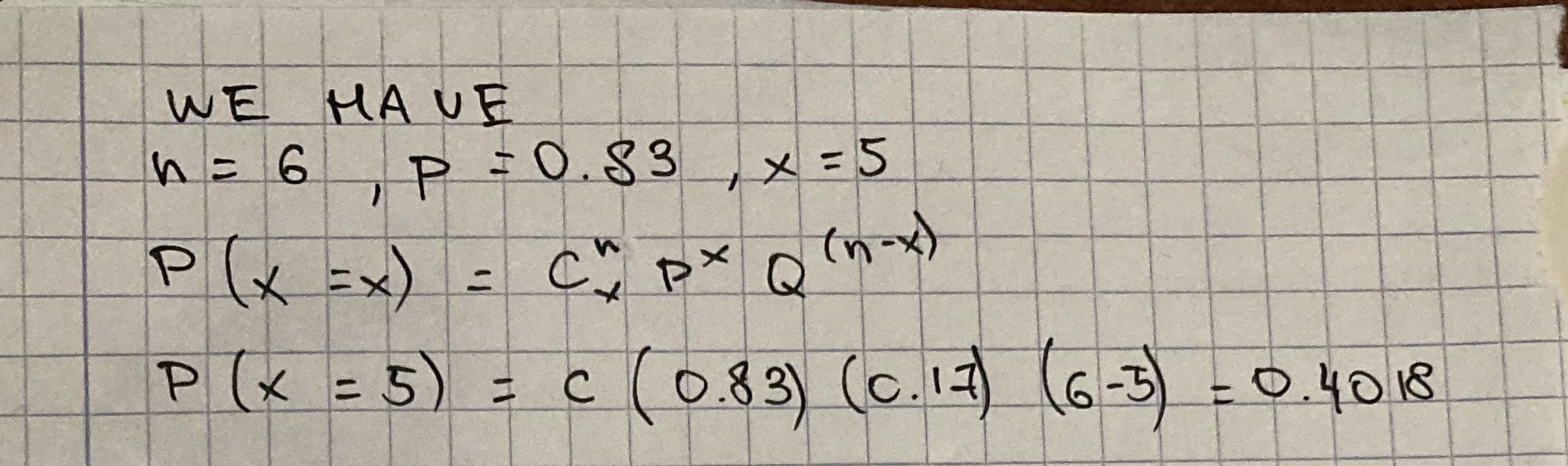Please, find the binomial probability when n = 6, p = 0.83, x = 5.shadsiei 2021-01-23 Answered

Please, find the binomial probability when $$n = 6, p = 0.83, x = 5.$$

• Questions are typically answered in as fast as 30 minutes

Solve your problem for the price of one coffee

• Math expert for every subject
• Pay only if we can solve itSally Cresswell.